American Journal of Theoretical and Applied Statistics
Volume 4, Issue 6, November 2015, Pages: 619-629

Estimation of Proportion of a Trait by Batch Testing Model in a Quality Control Process

Ronald W. Wanyonyi1, Kennedy L. Nyongesa2, Adu Wasike2

1Department of Mathematics, Egerton University, Nakuru, Kenya

2Department of Mathematics, Masinde Muliro University of Science and Technology, Kakamega, Kenya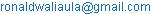(R. W. Wanyonyi)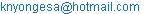(K. L. Nyongesa)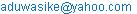(A. Wasike)

Ronald W. Wanyonyi, Kennedy L. Nyongesa, Adu Wasike. Estimation of Proportion of a Trait by Batch Testing Model in a Quality Control Process. American Journal of Theoretical and Applied Statistics. Vol. 4, No. 6, 2015, pp. 619-629. doi: 10.11648/j.ajtas.20150406.34

Abstract: Batch testing involves testing items in a group rather than testing the items individually for resource saving purposes. Estimation of proportion of a trait of interest using batch testing model insulates individuals of a population against stigma. In this paper, an estimator of the unknown proportion of a trait in batch testing model based on a quality control process is constructed and its properties discussed. In quality control, a batch is rejected if constituent defective members are greater than l, the cut off value. It is observed that if l = 0, then the obvious batch testing strategy is obtained. Hence when l > 0, the batch testing strategy is generalized. The proposed model is superior to the existing models when the proportion of a trait is relatively high. The application of the model on Genetically Modified Organisms contamination is carried out.

Keywords: Quality Control, Batching Testing, Cut off Value, Proportion, Genetically Modified Organisms

1. Introduction

Batch testing, also known as pooled testing or group testing, has a rich history going back to  and it involves testing several items simultaneously in a batch. Since his seminal work, batch testing has been applied to problems in blood bank screening, genetics, drug discovery, epidemiology and quality control. In all these applications, batch testing has two main objectives; first is the identification of positive individuals in a large population [1,2,3]. Second is estimation of the proportion of character or trait of interest [4,5,6,7,8,9,10].

Recent studies in batch testing have concentrated on the second objective by generalizing  and  studies. The various scholars have done this by considering; unequal pool sizes [11,12], multistage pooling schemes [3,7,8], imperfect tests [9,13] and  introduced the idea of blockers. However, in both objectives, batch testing has been shown to offer substantial gains (compared to one-at-a time testing) when dealing with rare traits (i.e when proportion, p is small). Recent advances in batch testing have included considering regression models for batch testing and hierarchical batch testing. For example,  examined two-stage hierarchical batch testing for multiple infections in which they developed an expectation-maximization algorithm to estimate probabilities of infections using both batch and individual retest responses.  proposed retesting of random grouping of units from positive batches. They noted that this option is useful when tests are not destructive or acquisition of additional units is impractical or too expensive. An advance brought about by batch testing is the ability to assess significance of covariate effects and/or reporting subject-specific estimates of the probability of infection cost effectively . This is achieved by developing regression models for batch testing that exploit the information available from the underlying biomarker.

In many industrial applications in which acceptance sampling is employed, a batch is classified positive or negative according to whether the number of constituent members with the quality trait is greater or less than a fixed cut of value or threshold, l . But the quality control processes can be resource intensive especially when tests involved are expensive and/or takes longer to get the results. Therefore, substantial savings can be realized if batch testing is applied. In this paper we present an estimator of the unknown proportion of a trait in batch testing model based on a quality control process and its properties are discussed.

2. Point Estimation of p

Consider a finite population with N items and each item can be classified as good or defective and let p, be the unknown proportion of defective items in the population. Suppose that the population can be divided into n batches each of size k. A batch is rejected if the number of defective items, d in the batch is greater than a predefined threshold or cutoff value,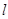. The probability of rejecting a batch expressed in terms of cumulative distribution function is;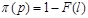(1)

It is noted that the relationship betweenand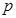is sensitive to both k and l as the slope of the curve changes with varying k and l.

Suppose X out of the n batches test positive on the test. Here X is a random variable and according to Dorfman (1943), X follows a binomial (n,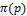). The MLE of p is obtained by solving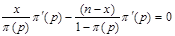(2)

where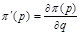and q = 1- p.

There are two possible solutions to Equation (2);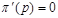or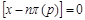The candidate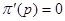is dropped because it gives only two extreme solutions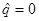or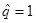and thus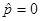or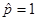. The estimateis most unlikely to happen since in practice it is not possible to avoid defectives and have only good items. On the other handmeans that all the batches or items test positive which is certainly an overestimate ofas it is most unusual for every item in the population to be positive (Hepworth and Watson, 2009). With the candidate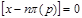, we have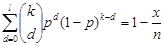(3)

Equation (3) has no solution in closed form except when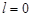, which leads to the results obtained by Thompson (1962) as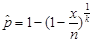(4)

Hence, the proposed estimator generalizes  results. When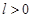, Equation (3) can be solved iteratively. The table below shows the MLE of p for different values of p, k and l.

Table 1. MLE of p for k = 5, 10, 15, 25 and l = 0, 1, 2, and 3.

 l p 0 1 2 3 k = 5 0.005 0.00101 0.02288 0.08283 0.18511 0.01 0.00198 0.03269 0.10564 0.22207 0.05 0.01022 0.07646 0.18924 0.34259 0.10 0.02085 0.11222 0.24663 0.41610 0.15 0.03198 0.14187 0.28990 0.46795 0.20 0.04364 0.16861 0.32659 0.50979 0.25 0.05588 0.19377 0.35944 0.54582 k = 10 0.005 0.00051 0.01085 0.03701 0.07678 0.01 0.00098 0.01554 0.04750 0.09321 0.05 0.00514 0.03679 0.08728 0.15000 0.10 0.01048 0.05456 0.11580 0.18756 0.15 0.01612 0.06949 0.13813 0.21563 0.20 0.02209 0.08326 0.15763 0.23944 0.25 0.02836 0.09640 0.17557 0.26085 k = 15 0.005 0.00035 0.00709 0.02388 0.04876 0.01 0.00065 0.01019 0.03074 0.05939 0.05 0.00344 0.02422 0.05687 0.09666 0.10 0.00699 0.03603 0.07585 0.12177 0.15 0.01078 0.04606 0.09087 0.14080 0.20 0.01477 0.05531 0.10408 0.15716 0.25 0.01899 0.06419 0.11634 0.17205 k = 25 0.005 0.00021 0.00423 0.01400 0.02824 0.01 0.00039 0.00604 0.01802 0.03446 0.05 0.00202 0.01439 0.03351 0.05656 0.10 0.00422 0.02148 0.04493 0.07166 0.15 0.00648 0.02748 0.05394 0.08324 0.20 0.00888 0.03309 0.06200 0.09328 0.25 0.01142 0.03849 0.06950 0.10243

From Table 1, we note that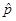increases as l increases for any fixed p and k and at the same timereduces as k increases for fixed p and l. Thus, it is possible to geta combination of k and l that gives closer to a fixed p. We investigated the relationship betweenandfor different values of k and l as presented by Figure 1 below.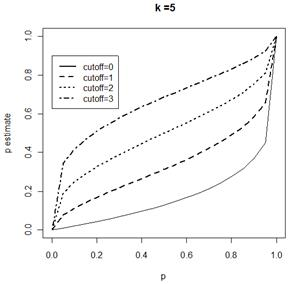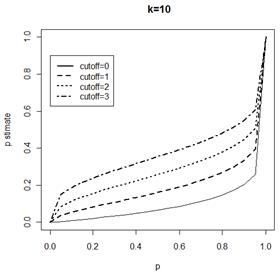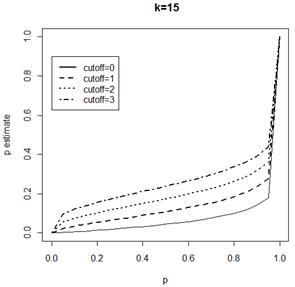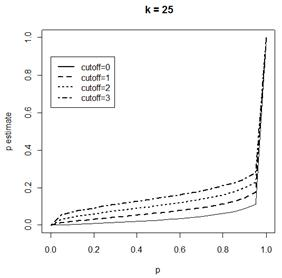Figure 1. Plots of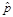against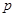for k = 5, 10, 15, 25 and l = 0, 1, 2 and 3.

The relationship betweenandin batch testing when l = 0 has been investigated by . It was found that there is a linear relationship betweenandfor k = 1. Figure 1 clearly shows the effects of k and l on the estimate of the proportion. The relationship betweenandis found to be monotonic but not linear as opposed to the case when batch size is equal to 1. It is also noted that the relationship is sensitive to both k and l.

3. Properties of the Estimator

In this section, the properties of the estimator are discussed.

3.1. Asymptotic Variance

The asymptotic variance is obtained from the Fisher’s information given by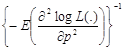(5)

which gives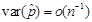(6)

Derivation of Equation (6) is provided in the Appendix A. This asymptotic variance can be used to construct the Wald-type confidence intervals for the proportion of defectives. To investigate the behavior of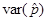, we have plottedagainstfor different k and l.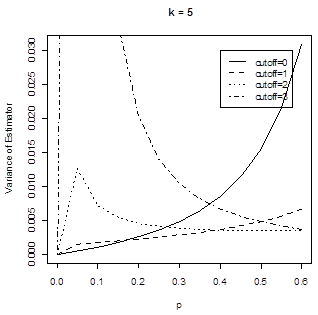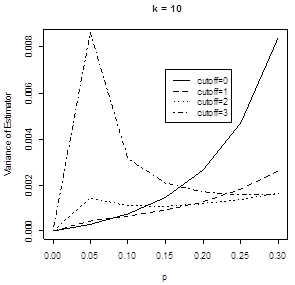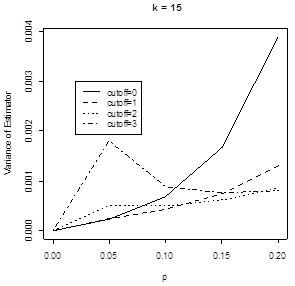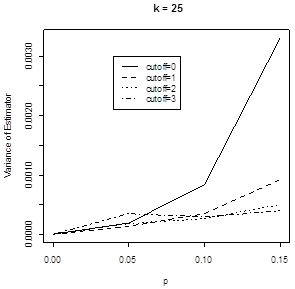Figure 2.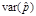as a function of p for k = 5, 10, 15, 25 and l = 0, 1, 2 and 3.

The principal interest in Figure 2 is to observe where the curves for l > 0 lie below the curve for l = 0 i.e. the solid line. It indicates that the model with l > 0 has low variance for fairly higher proportions and this is achieved at lower p as k increases.

Next, we compare our results with those of  among others by computing Asymptotic Relative Efficiency (ARE). If the estimator of  among others is denoted by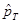and our estimator is denoted by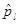, then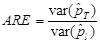(7)

Therefore, ARE > 1 implies that the proposed model is more efficient than the  model. The computed ARE is plotted against the p for various k and l as shown in Figure (4) below.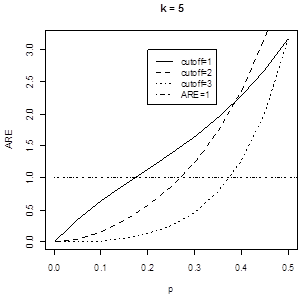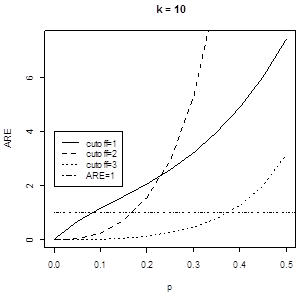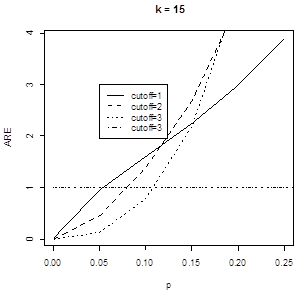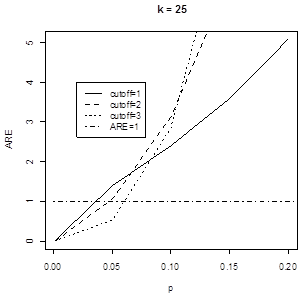Figure 3. ARE plotted against p for k = 5, 10, 15, 25 and l = 1, 2 and 3.

The proposed model is more efficient than the Thompson estimation model for relatively higher p. However, the p for which the proposed model is more efficient than Thompson’s model reduces as k increases. Therefore, the proposed model performs better in situations where p is relatively high which has been a drawback to the use of batch testing strategy.

3.2. Bias of the Estimator

The bias is used to measure the average error incurred when using the estimate of a parameter. The bias of an estimator is given by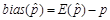(8)

and if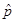follows binomial distribution with parameters (n,), then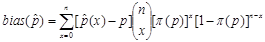(9)

This might not be the case in practice as assumed by  and  among others and hence we invoke Monte Carlo simulations.

Since the bias of an estimator is related to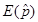, we also investigated the behavior of bias by plottingagainst p for different k and l as shown below. Thewhen k = 1 is represented by a solid line in the Figure 4.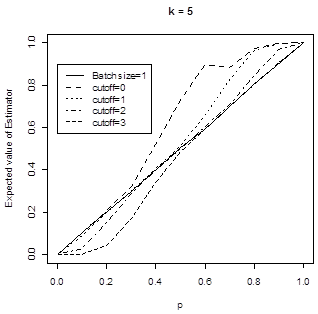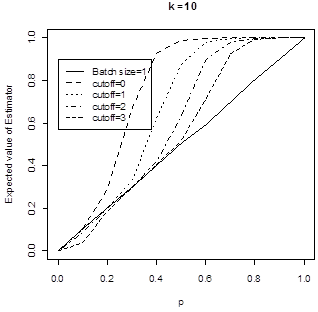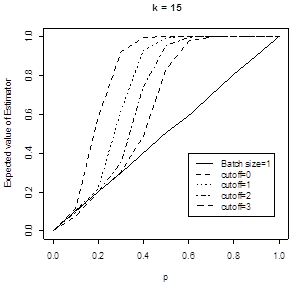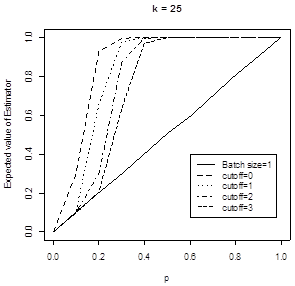Figure 4.as a function of p for l = 0, 1, 2 and 3 with k = 5, 10, 15 and 25.

The expected values of the estimator is strongly dependent on l. Note also that when l = 0, the estimator is positively biased. That is, the estimator overestimates p as earlier noted by . Since then the batch testing literature has continued to note this fact [20,21] or indicated how it can be derived by approximate expression using Taylor series expansion  or by help of Jensen’s Inequality . On the other hand, when l > 0 the estimator underestimates p for low p < 0.5 but overestimates p for higher p. The amount of bias is noted to be affected by the cut off value and the bias is negligible when the p is low.

Overall, the first thing noted is that choosing k too large must be avoided. For example, if p = 0.3, the ideal batch size would be k = 5 or 10 since the bias is negligible for cut off values l = 1, 2 and 3. Secondly, even when p is quite large (up to p = 0.7) there is k for which the bias is negligible. Lastly, since in practice the p is likely to be small, it should be easy to choose k and l for which the bias is negligible.

3.3. Mean Squared Error of the Estimator

The Mean Squared Error (MSE) of an estimator is the expected value of the squared difference between the unknown parameter and its estimator and is used as a measure for the goodness of an estimator. Swallow (1985) gives MSE of the estimator of p to be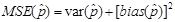(10)

From Equation (9), the MSE amalgamates the information from both the bias and the variance of the estimator and will be inflated by either large bias (inaccuracy of the estimator) or large variance (poor precision). The main goal of batch testing in the estimation problem is to minimize the MSE of the estimator of p as mentioned in Hung and Swallow (1999). The figure that follows shows the MSE of the estimator versus p for different values of l and k obtained by simulation. The solid line represents the MSE when k = 1.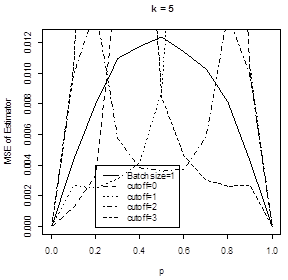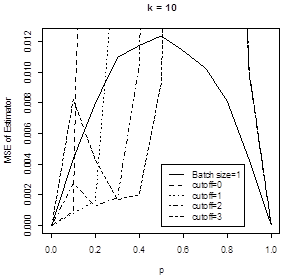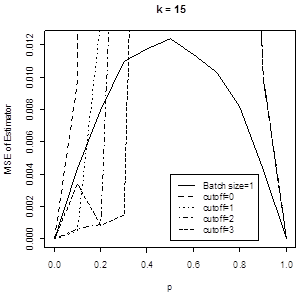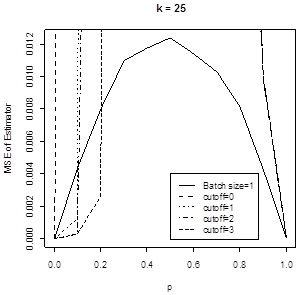Figure 5. MSE of the estimator plotted against p for l = 0, 1, 2 and 3 with k = 5, 10, 15 and 25.

Figure 4 prompts several comments. With k = 5 and p < 0.2, batch testing using l = 0 or 1 can greatly reduce the MSE of the estimator. Further, classical batch testing has low MSE for low values of p < 0.18 than batch testing with l = 1. For higher p > 0.2 batch testing with l > 0 is better than the classical batch testing and for p > 0.6 batch testing with l = 3 is better than batch testing with lower l < 3. When the batch size is increased to k = 10, the batch testing with l > 0 is better than the classical batch testing for p > 0.1. Also it can be noted that for fairly large values of p > 0.3, the batch testing with larger l = 3 would be preferred to those using small l < 3.

With k = 15, batch testing with l > 0 has low MSE compared to classical batch testing except for very low values of p. The model with l = 3 however performs better than others when p > 0.2.

When k = 25 the batch testing with l =3 has low MSE compared to others when p > 0.1. Generally, batch testing with l = 3 performs better than batch testing with l < 3 for high p. But it is also worth to note that these values of p for which the model with l =3 performs better than others reduces as k increases. For example when k = 5 that value is p > 0.6 while when k = 10 the value reduces drastically to p > 0.1. If the number of batches or assays may be limited by cost, say but the number of items in a batch can be chosen without any restrictions. Then k becomes an issue to be considered in order to get the best estimator. It is noted (results not included) that k for which the model performs efficiently is much less when p is high as opposed when p is low. Therefore, the choice of k also depends on p.

The RMSE is a convenient measure for comparing the MSEs of estimates of the same p that is obtained by using different experimental designs or procedures. In this case the estimator using the proposed model is compared with that of  among others. The RMSE is calculated as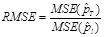(11)

Table 2. RMSE for various values of p with l = 1, 2, 3 and k = 5, 10, 15 and 25.

 l p 1 2 3 k = 5 0.005 1.0808780 2.1792380 2.17923800 0.01 0.5691428 0.7733947 1.02110600 0.05 0.2726298 0.1981342 0.22624370 0.10 0.4952901 0.1352474 0.12972640 0.15 0.7832783 0.1321585 0.08409845 0.20 1.3067560 0.2421896 0.08576563 k = 10 0.005 0.6667864 1.0734130 1.0734130 0.01 0.3709624 0.4818073 0.5383903 0.05 0.5189823 0.1530551 0.1324795 0.10 1.3166490 0.3408400 0.1101625 0.15 8.6156060 5.3238890 0.9764622 0.20 30.0809600 48.1317600 14.7718900 k = 15 0.005 0.4225163 0.6025715 0.7310380 0.01 0.3043926 0.3213549 0.3619061 0.05 1.0201640 0.2010700 0.1133701 0.10 18.7554200 12.5215900 2.4391450 0.15 137.420600 161.737700 94.988850 0.20 17.037750 313.928900 364.764500 k = 25 0.005 0.3358621 0.4108387 0.4626208 0.01 0.3078288 0.2146209 0.2359505 0.05 7.638219 3.565189 0.8637323 0.10 160.7329 681.5068 604.3566 0.15 4.600869 123.6423 1282.223 0.20 1.534643 6.501305 128.6813

The results show that for small k = 5, the proposed model performs well compared to Thompson’s model for low value of p = 0.005 and higher value of p = 0.20. This is indicated by the value of RMSE > 1. As k increases, the model becomes more efficient than Thompson’s model for higher values of p. For instance, if p = 0.05 and k = 25, the proposed model with l = 1 is 7.6 times more efficient than the Thompson’s model.

4. Application of the Model

The model developed can be applied in the case of checking for the quality of produced items before being released to the customers or incoming products by organizations. In checking for quality, a batch of the product is accepted if the number of defectives as far as the trait of interest is less than a predefined tolerance level or cut off. This happens in seed production . The concerned organizations normally design what is called testing plan which has two key parameters; the number of individual items to sample and test and the maximum number of defectives or unacceptable items (or batches) that can be tolerated in the sample before the batch is rejected.

In the case of commercial seed production, unintentional mingling of GMOs with non-GMOs has attracted a lot of public attention. Therefore, there is important need to control unauthorized GMOs existing in the commercialized batches of seeds or when legislation makes the labeling compulsory that is has to be adequately labeled. The procedures employed in detecting the presence of GMOs use ELISA or PCR methods which have threshold of detection below which the existence of the trait of interest is not detected.

It is worth to indicate here that in practice, three situations are encountered:

1.  The number of items to be tested is unlimited but the number of batches formed is restricted by the cost of the testing procedure (assays).

2.  The batch size might be limited by the dilution effect of the items being tested leading to misclassification errors.

3.  There are cases where the total number of items to be tested is limited.

The model developed in this study is applied to the three situations mentioned above:

Example 1

This example was reported by  but with a few modifications; reducing batch size and assuming that the quantitative technique was applied in testing conventional seeds for the presence of GMOs. In the experiment, 6300 seeds were assigned to 126 batches each containing 500 seeds. The threshold of detection is 0.005 which means a batch of size 500 is rejected if the number of GMO seeds is greater than 3. The results are represented below:

Table 3. Estimates for p with n and k fixed and varying X.

 Case 1 Case 2 Case 3 Case 4 Case 5 Case 6 l 3 3 3 3 3 3 n 126 126 126 126 126 126 X 21 24 27 30 31 32 k 500 500 500 500 500 500 MLE 0.00424 0.00450 0.00476 0.00497 0.00501 0.00514 Bias (x10-6) 57 7 5 3 6 4 MSE (x10-7) 2.6165 1.2515 1.2655 1.2642 1.2646 1.2760 95% lower limit 0.003542 0.003830 0.004105 0.004241 0.004241 0.004439 95%upper limit 0.004907 0.005176 0.005496 0.005655 0.005655 0.005816 Length of 95% CI 0.001365 0.001346 0.001391 0.001414 0.001414 0.001377

In this Example 1, X was increased but maintaining the same l, n and k. The results indicate several characteristics of the MLE. As X increases, the MLE also increases. This is expected because a higher X indicates higher proportion of defective items in the population. The bias of the estimate reduces as X increases and a minimum bias is observed when X is equal to 30. However, the minimum MSE of estimate and length of confidence interval is obtained when X is equal to 24. The 95% CI for the proportion, p in case1 does not contain the threshold proportion (0.005) and the conclusion is that in this case the concerned batch contains less than the threshold proportion at 95% confidence level. However, in other cases the 95% CI for the proportion contains the threshold proportion and thus if given population has 24 or more positive batches on testing then it should be rejected or labeled accordingly at 95% confidence level.

The number of batches and the batch size in this example is for the investigation of the properties of the MLE of the proposed model. But the batch size is very close to the one recommended by GIPSA in testing seeds for GMO which is 400 seeds .

Example 2 (Hypothetical situation)

The second example also concerns the procedure of detecting GMOs in commercial seed production and the unit of sampling is one seed. Both ELISA and PCR are used in the detection of GMOs with threshold of detection 0.005. The example presents different scenarios; varying k and X.

Table 4. Estimates of p with n is fixed and varying k and X.

 Case 1 Case 2 Case 3 Case 4 Case 5 Case 6 Case 7 Case 8 Case 9 l 1 2 4 1 2 4 1 2 4 n 20 20 20 20 20 20 20 20 20 X 1 1 1 5 5 5 10 10 10 k 100 400 800 100 400 800 100 400 800 MLE 0.00357 0.00247 0.00205 0.00961 0.00432 0.00421 0.01673 0.00668 0.00584 Bias (x10-4) 8.14 2.68 9.64 10.00 0.66 6.10 3.34 0.69 0.19 MSE (x10-6) 7 1 3 8 1 0.57 14.00 1.30 0.58 95% lower limit 0 0 0 0.005333 0.002761 0.003014 0.01097 0.004777 0.004542 95% upper limit 0.006848 0.003846 0.003014 0.015206 0.006175 0.005509 0.02422 0.009039 0.007333 Length 95% CI 0.006848 0.003846 0.003014 0.009873 0.003414 0.002495 0.01325 0.004262 0.002791

For this example, in case 1, case 2 and case 3, k was increased while keeping n = 20 and, X = 1 while in case 4, case 5 and case 6, X = 5. Lastly, case 7, case 8 and case 9 the positive batches was fixed at X = 10. The results show that the MLE reduces with increasing k in all the cases. In all cases, the length of the 95% CI decreases with increasing k. This is in accordance with the law of large numbers which assures that the estimate of p converges to p as k increases. From the results, it is also noted that as n increase the length of the confidence interval increases. The bias of the estimate increased with k but reduced with the increase in X. However, the MSE of the estimate did not exhibit a simple kind of relationship between k and X.

Example 3

In the control of GMO seeds in commercial seeds, Grain Inspection, Packers and Stockyards Administration (GIPSA) recommends acceptance sampling plans using control by attributes and has been adopted by several seed producers and grain exporters . The sampling plan was designed such that the producer’s and consumer’s risks are minimized. The standard sample size that achieves this was found to be 2400 seeds per batch.

Now performing a single test on the sample may not be practical as it could lead to higher rate of misclassifications due to dilution effect of test material. On the other hand, testing individual seeds leads to wastage of resources especially expensive assays involved. Thus, the 2400 seeds are normally divided into batches of size 400 seeds and each batch tested. The threshold in this case is 1% and so a pool is adjudged to be positive if the GMO seeds in the batch are greater than 4. The results are recorded below:

Table 5. Estimates of p in GIPSA recommended acceptance sampling plan.

 Case 1 Case 2 Case 3 Case 4 Case 5 l 4 4 4 4 4 n 6 6 6 6 6 X 1 2 3 4 5 k 400 400 400 400 400 MLE of p 0.00724 0.00952 0.01167 0.01412 0.01761 Bias of estimate 0.00177 0.00151 0.01883 0.09058 0.30829 MSE of estimate 0.00002 0.00197 0.01857 0.08942 0.30401 Two-sided 95% lower limit 0 0 0.007228 0.009538 0.011694 Two-sided 95% upper limit 0.01169 0.014098 0.018267 1.000000 1.000000 Length of 95% CI 0.01169 0.014098 0.011039 0.990462 0.988306

The MLE of p and MSE of the estimate both increase as X increases. The bias of the estimate attains the minimum at the point when X is equal to 2. But the minimum length of the confidence interval occurs when X is 3.

5. Discussion

Batch testing procedure where batches are classified as positive if they contain more than l defective items has been presented and its likelihood estimator for the proportion of a trait derived. This procedure is usually used in quality control by organizations before procuring or releasing a product to the market to cut on costs. We derived the MLE of p and found that it generalizes the estimator obtained by . It is also seen that our estimator has no closed form solution when l > 0 and hence R-code, using in-built function uniroot, is developed to determine the estimates for various values of p, k and l. From Table 1 and Figure 1, it is noted that the MLE of p monotonically increases with p but the relationship is not linear and greatly affected by both k and l. As it can be observed from Figures 3 and 5, the method improves the efficiency of the estimator over the classical batch testing for relatively high proportions. But the efficiency of the estimator can be improved for low proportions by increasing the sample size. It is further observed that the estimator is negatively biased for proportion when sample size is small and proportion low but positively biased for large proportions. But for high proportions the estimator is positively biased for proportions.

We also considered the behavior of the estimator when the proposed model is applied in screening for the presence of GMO in seeds. Note that he procedure used in testing for GMO in seeds is well detailed by . The paper looks at three scenarios are commonly encountered in practice; number of batches is limited, batch size is restricted and number of units to be tested is limited. In all situation, the study found that we can set k and l so as to obtain an estimate of the given p with minimum MSE or bias.

This paper has considered a case in which the tests are assumed to be perfect and the detection of defective unit can be accurately determined but this may not be the case in most applications. In fact  noted that ELIZA assay has sensitivity of 97.7% and specificity of 92.6%. Therefore, we propose the examining of batch testing based on quality control processes with imperfect tests. That is, these tests have sensitivity and specificity less 100% and thus lead to misclassifications (false positive or false negative). Also, it is worthy considering the case where retesting of positive batches is allowed since retesting can make up for lost sensitivity due to pooling in first stage.

Appendix A: Derivation of Asymptotic Variance

The asymptotic variance is computed by solving;But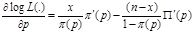and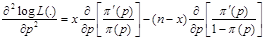(12)

Thus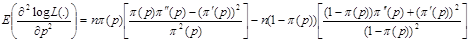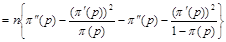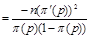(13)

hence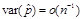References

1. Dorfman, R. (1943). The detection of defective members of large population. Annals of Matematical Statistics, 14,436-440.
2. Johnson, N. I., Kotz, S. and Wu, X. (1991). Inspection errors for Attributes in Quality Control. London; Chapman and Hall.
3. Nyongesa, L. K. (2004). Testing For the Presence of Disease by Pooling Samples. Aust. N. Z. J. Stat., 46, 901 -909.
4. Thomson, K. H. (1962). Estimation of the Proportion of Vectors in a Natural Population of Insects, Biometrics, 18, 568-578.
5. Sobel, M., and Elashoff, R.M., (1975). Group -testing with a new goal, Estimation. Biometrika, 62, 181-193.
6. Gastwirth, L. J. and Johnson, W. O. (1994). Screening with Cost-Effective Quality Control: Potential Applications to HIV and Drug Testing. Journal of the American Statistical Association, 89, 972-981.
7. Brookmeyer, R. (1999). Analysis of Multistage Pooling Studies of Biological Specimens for Estimating Disease Incidence and Prevalence. Biometrics, 55, 608-612.
8. Hughes-Oliver, M. J. and Swallow, H. W. (1994). A Two-Stage Adaptive Procedure for Estimating Small Proportions. Journal of the American Statistical Association, 89, 982-993.
9. Tu, M. X., Litvak, E. and Pagano, M. (1995). On the Informative and Accuracy of Pooled Testing in Estimating Prevalence of a Rare Disease: Application to HIV Screening. Biometrika, 82, 287-297.
10. Xie, M., Tatsuoka, K., Sacks, J. and Young, S.S. (2001). Group Testing With Blockers and Synergism. Journal of the American statistical association, 96, 92-102.
11. Hepworth, G. (1999). Estimation of Proportions by Group Testing. PhD thesis, University of Melbourne.
12. Biggerstaff, B. (2008). Confidence Intervals for the Difference of two proportions Estimated from Pooled samples. Journal of Agricultural, Biological and Environmental Statistics, 13, 478-496.
13. Nyongesa, L. K., (2011). Dual Estimation of Prevalence and Disease Incidence in Pool-Testing Strategy. Communication in Statistics Theory and Method. 40, 1-12.
14. Tebbs, J. M., McMaham, C. S. and Bilder, C. R. (20130. Two-stage Hierarchical Group Testing for Multiple Infections with Application to the Infertility Prevention Project, Biometrics, 69, 1064-1073.
15. Hepworth, G. and Watson, R. (2015). Revisiting Retesting in the Estimation of Proportions by Group- Testing, Communications in Statistics-simulation and Computation, DOI:10.1080/03610918.2014.960725.
16. McMaham, C. S., Tebbs, J. M. and Bilder, C. R. (2013). Regression Models for Group Testing Data with Pool Dilution Effects, Biostatistics, 14, 284-298.
17. Montegmery, D. C. (2009). Introduction to Statistical Quality Control (6th edition). New York, John Wiley and Sons.
18. Hepworth, G. and Watson, R. (2009). Debiased Estimation of Proportions in Group Testing. Applied Statistics, 58, 105-121.
19. Schaarschmidt, F. (2007). Experimental Design for One-sided Confidence Intervals or Hypothesis tests in Binomial Group Testing. Communications in Biometry and Crop Science, 2, 32-40.
20. Bhattacharyya, G. K., Karandinos, M. G., and DeFoliart, G. R. (1979). Point Estimates and Confidence Intervals for Infection Rates Using Pooled Organisms in Epidemiologic Studies. American Journal of Epidemiology, 109, 124-131.
21. Swallow, W. H. (1985). Group Testing for Estimating Infection Rates and Probability of Disease Transmission. Phytopathology, 75, 882-889.
22. Lovison, G., Gore, S. D. and Patil, G. P. (1994). Design and Analysis of Composite Sampling Procedures: a review. In Handbook of Statistics, 12, 103-166. Amsterdam: Elsevier.
23. Hung, M. and Swallow, H. W. (2000). Use of Binomial Group Testing In Tests of Hypotheses for Classification or Quantitative Covariates. Biometrics, 56, 204-212.
24. Remund, K. M., Wright, D. L. and Holden, L. R. (2001). Statistical Considerations in Seed purity testing for transgenic traits. Seed Science Research, 11, 101-120.
25. Kobilinsky, A. and Bertheau, Y. (2005). Minimum Cost Acceptance sampling plans for Grain Control with application to GMO detection. Chemometrics and Intelligent Laboratory Systems, 75, 189-200.
26. Montesinos-Lopes, O. A., Montesinos, A., Eskridge, K. and Crossa, J. (2014). Estimating a Proportion based on Group Testing for Correlated Binary Response. J Biomet biostat, 5, 185-196.
27. Liu, C., Liu, A., Zhang, B. and Zhang, Z. (2013). Improved Confidence Intervals of a small Probability from Pooled Testing with Misclassification. Frontiers in Public Health, DOI: 10.3389/fpubh.2013.00039.

 Contents 1. 2. 3. 3.1. 3.2. 3.3. 4. 5.
Article ToolsAbstractPDF(346K)